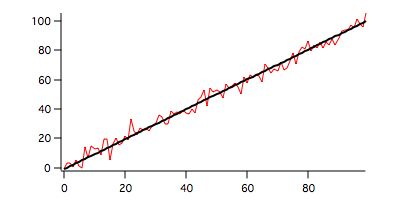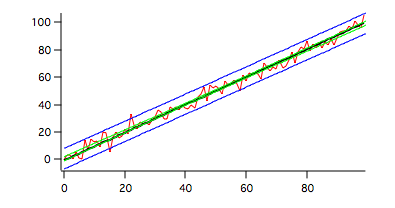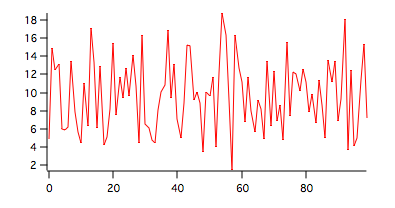You can perform (linear) regression analysis using Igor's curve fitting operations.. StatsLinearRegression provides more statistical information/tests for single and multiple regressions.

1. Simple linear regression.

Start by creating a wave with a known slope and additive Gaussian noise.

`Make/O/N=100 data1=x+gnoise(4)`

The simple linear regression analysis is obtained by:

`StatsLinearRegression /T=1/Q  data1`

The results appear in the Linear Regression table (shown transposed):

 N 100 a -0.912472 b 1.01654 xBar 49.5 yBar 49.4064 sumx2 83325 sumy2 87529 sumxy 84703.4 Syx 3.81255 F 5923.74 Fc 3.93811 r2 0.983726 Sb 0.0132077 t tc 1.98447 L1 0.990332 L2 1.04275

When a slope is not specified the null hypothesis is that the slope is zero and the t-statistic is not relevant. The regression results above clearly reject this hypothesis with regression line given by:

`y=-0.912472+1.01654*x.`

To plot the regression and the data curves execute the commands:

```Duplicate/O data1,regression1
regression1=-0.912472+1.01654*x
Display/K=1 data1,regression1
ModifyGraph lsize(regression1)=2,rgb(regression1)=(0,0,0)
```Here the red trace corresponds to our input data and the black trace is the regression line.

You can create confidence interval waves and prediction interval waves using

`StatsLinearRegression /T=1/Q/BCIW/BPIW  data1`

You can now add the two bands to the graph:

```AppendToGraph data1_CH,data1_CL,data1_PH,data1_PL
ModifyGraph rgb(data1_CH)=(0,65535,0),rgb(data1_CL)=(0,65535,0)
ModifyGraph rgb(data1_PH)=(0,0,65535),rgb(data1_PL)=(0,0,65535)
```Here the green traces correspond to the confidence interval while the blue traces correspond to the prediction interval (default single prediction).

2. Zero slope hypothesis

To see the results of the zero-slope hypothesis test on different data set we generate data that have zero slope and random noise:

`Make/O/N=100 data2=10+gnoise(4)`A graph pf data2 is shown above. To run the test execute the command:

`StatsLinearRegression /T=1/Q  data2`
 N 100 a 9.44344 b 0.00449769 xBar 49.5 yBar 9.66608 sumx2 83325 sumy2 1505.25 sumxy 374.77 Syx 3.91695 F 0.109865 Fc 3.93811 r2 0.00111981 Sb 0.0135694 t tc 1.98447 L1 -0.0224303 L2 0.0314257

As expected, F<Fc and the hypothesis of zero slope must be accepted.

3. Specific slope hypothesis

Testing the hypothesis that the slope b=beta0. Using the wave data1 which was generated with a unit slope we have:

`StatsLinearRegression /T=1/Q/B=1.0  data1`
 N 100 a -0.912472 b 1.01654 xBar 49.5 yBar 49.4064 sumx2 83325 sumy2 87529 sumxy 84703.4 Syx 3.81255 F 5923.74 Fc 3.93811 r2 0.983726 Sb 0.0132077 t 1.25247 tc 1.98447 L1 0.990332 L2 1.04275

It should be obvious from the definitions of L1 and L2 that any value of beta0 outside the range [L1,L2] will be rejected.

4. Linear regression for more than one wave.

```Make/O/N=100 data3=4+x+gnoise(4)
Make/O/N=100 data4=5+x+gnoise(5)
```

You can run the linear regression test on multiple samples using the command:

`StatsLinearRegression /T=1/Q data1,data3,data4`

The results are displayed in the Linear Regression table and in the Linear Regression MC table.

Linear Regression table:

 data data3 data4 N 100 100 100 a -0.912472 4.10709 4.37794 b 1.01654 0.993232 0.993211 xBar 49.5 49.5 49.5 yBar 49.4064 53.272 53.5419 sumx2 83325 83325 83325 sumy2 87529 83725. 85298.4 sumxy 84703.4 8276 82759.3 Syx 3.81255 3.94368 5.62508 F 5923.74 5285.34 2597.77 Fc 3.9381 3.9381 3.93811 r2 0.983726 0.981796 0.963647 Sb 0.0132077 0.013662 0.0194868 t tc 1.98447 1.98447 1.98447 L1 0.990332 0.96612 0.95454 L2 1.04275 1.02034 1.03188

Linear Regression MC table:

 Ac 249975 Bc 250224 Cc 256552 SSp 6049.51 SSc 6079.72 SSt 7150.35 DFp 294 DFc 296 DFt 298 Slopes F 0.734116 Slopes Fc 3.02647 CoincidentalRegression F 13.375 CoincidentalRegression Fc 2.40235 Elevations F 26.0627 Elevations Fc 3.02626

In this case the slopes' test results in F<Fc so the hypothesis of equal slopes is accepted while elevation's test results F>Fc implies that the equal elevations hypothesis is rejected as is the possibility of coincidental regression.

You can also test waves of unequal lengths as in the following example:

```Make/O/N=150 data5=x+gnoise(4)
Make/O/N=100 data6=x+gnoise(5)
StatsLinearRegression /T=1/Q data1,data5,data6
```
 Ac 447888 Bc 449403 Cc 457496 SSp 6549.12 SSc 6571.72 SSt 6614.3 DFp 344 DFc 346 DFt 348 Slopes F 0.593541 Slopes Fc 3.02197 CoincidentalRegression F 0.855888 CoincidentalRegression Fc 2.3979 Elevations F 1.12087 Elevations Fc 3.02182

In this case both the slopes and the elevations are determined to be the same. The single test for coincidental regression would also indicate that the three waves have coincident regressions.

5. Dunnett's multi-comparison test for elevations.

You can perform Dunnett's multi-comparison test on the elevations of the several waves. In this example we define the first input wave as the control sample (/DET=0):

`StatsLinearRegression /T=1/Q/DET=0 data1,data3,data4,data5,data6`

The operation computes the linear regression and the general multi-comparison as described above. In addition it displays Dunnett's MC Elevations table for tests of each input wave against the control wave:

 Pair SE q qp Conclusion 1_vs_0 0.641955 6.02176 2.16539 0 2_vs_0 0.641955 6.44208 2.16539 0 3_vs_0 0.615424 0.127039 2.16539 1 4_vs_0 0.641955 1.23037 2.16539 1

The table indicates that the equal elevation hypothesis is rejected for the pairs data3-data1 and data4-data1. The hypothesis is accepted for the other combinations.

6. The Tukey test for multiple regressions

The test depends on the F tests for the slopes. If the slopes are determined to be the same then the Tukey test compares elevations. Otherwise, the Tukey test performs a multi-comparison of slopes.

`StatsLinearRegression /T=1/Q/TUK data1,data3,data4,data5,data6`

Since the slopes were determined to be the same, the Tukey test was performed on the elevations and the results are displayed in the Tukey MC Elevations table:

 Pair SE q qc Conclusion 4_vs_0 0.45393 1.7400 3.87073 1 4_vs_ 0.45393 6.77604 3.87073 0 4_vs_2 0.45393 7.37047 3.87073 0 4_vs_3 0.43517 1.63536 3.87073 1 3_vs_0 0.43517 0.17966 3.87073 1 3_vs_ 0.43517 8.7035 3.87073 0 3_vs_2 0.43517 9.32356 3.87073 0 2_vs_0 0.45393 9.11048 3.87073 0 2_vs_ 0.45393 0.594429 3.87073 1 1_vs_0 0.45393 8.51605 3.87073 0

As expected input waves 1 and 2 do not match the elevations of the other waves.

7. Regression analysis with multiple Y values for each X value

When the data has multiple Y values for each X value you need to store the input as a 2D wave there each column represents one set of Y values. In this example the wave dataMYV consists of 30 rows and 6 columns so there are at most 6 Y values for each X. There are 6 NaNs representing missing values or padding used to format the input for the operation in case the number of Y-values is not the same for all x values. The X-values are implied by the wave scaling of the rows with start=1 and delta=3.

`StatsLinearRegression /T=1/Q/MYVW={*,dataMYV}`

The results are displayed in the "Linear Regression" table (shown transposed):

 N 174 a -0.564705 b 2.01551 xBar 44.2586 yBar 88.639 sumx2 117111 sumy2 485408 sumxy 236039 amongGroupsSS 477052 amongGroupsDF 29 withinGroupsSS 8355.47 withinGroupsDF 144 devLinearitySS 1312.81 devLinearityDF 28 F 0.808045 Fc 1.55463 F2 8463.47 F2c 3.89609 r2 0.980082 Syx 7.4974

The F value is smaller than the critical Fc which implies that the regression of the data is indeed linear. The value of F2 is much greater than the critical value F2c which implies that the hypothesis of slope b=0 has to be rejected.ForumSupportGallery